# 3D Symmetric HO in Spherical Coordinates *

We have already solved the problem of a 3D harmonic oscillator by separation of variables in Cartesian coordinates. It is instructive to solve the same problem in spherical coordinatesand compare the results. The potential isOur radial equation is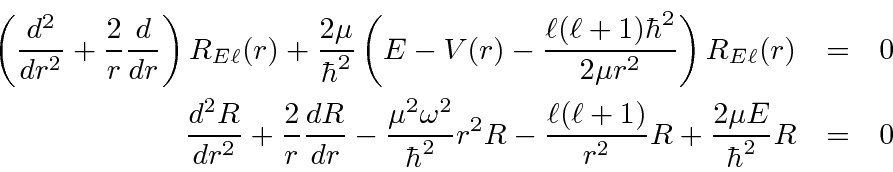Write the equation in terms of the dimensionless variable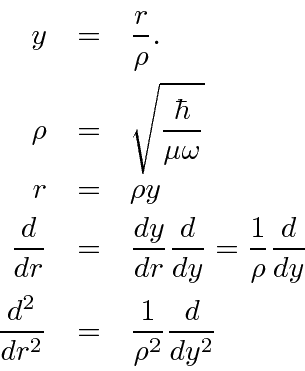Plugging these into the radial equation, we get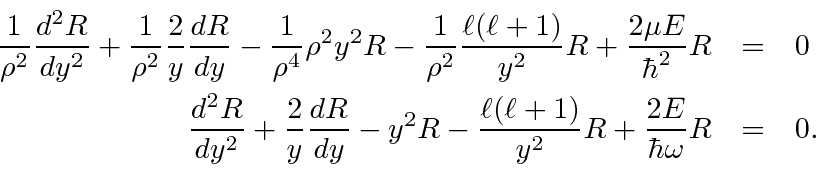Now find the behavior for large.Also, find the behavior for small.Explicitly put in this behavior and use a power series expansion to solve the full equation.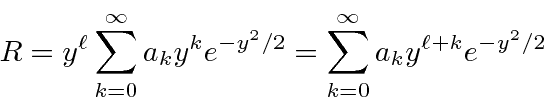We'll need to compute the derivatives.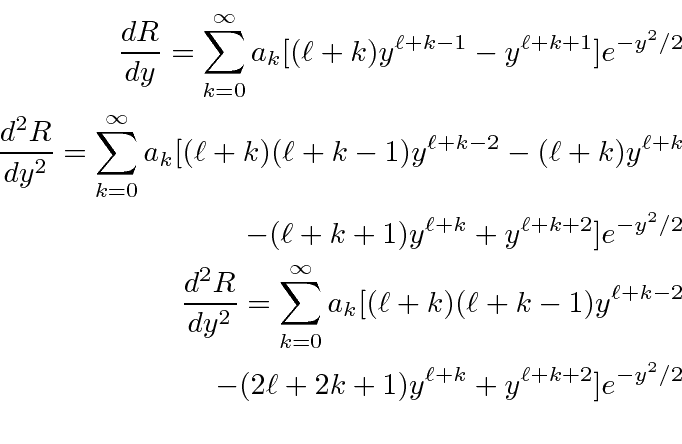We can now plug these into the radial equation.Each term will contain the exponential, so we can factor that out. We can also run a single sum over all the terms.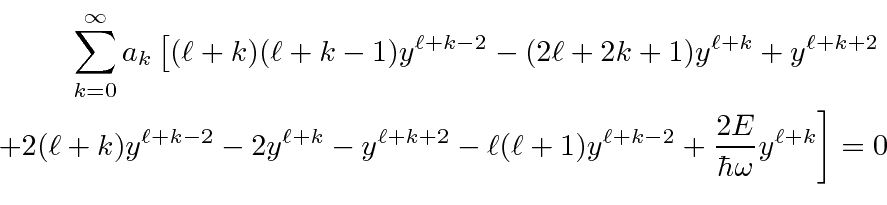The terms for largewhich go likeand some of the terms for smallwhich go likeshould cancel if we did our job right.Now as usual, the coefficient for each power ofmust be zero for this sum to be zero for all. Before shifting terms, we must examine the first few terms of this sum to learn about conditions on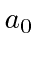and. The first term in the sum runs the risk of giving us a power ofwhich cannot be canceled by the second term if. For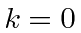, there is no problem because the term is zero. For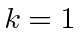the term iswhich cannot be made zero unlessThis indicates that all the odd terms in the sum will be zero, as we will see from the recursion relation.

Now we will do the usual shift of the first term of the sum so that everything has a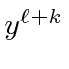in it.For large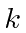,Which will cause the wave function to diverge. We must terminate the series for some, by requiringThese are the same energies as we found in Cartesian coordinates. Lets plug this back into the recursion relation.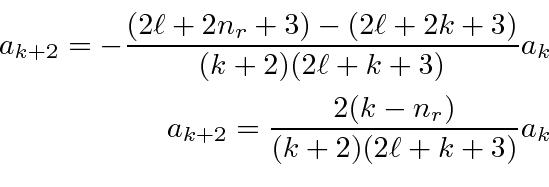To rewrite the series in terms of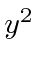and lettake on every integer value, we make the substitutions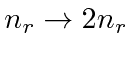and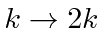in the recursion relation forin terms of.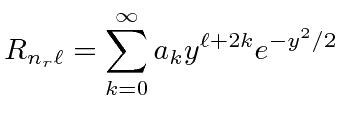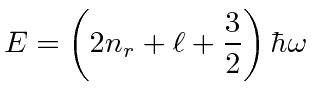The table shows the quantum numbers for the states of each energy for our separation in spherical coordinates, and for separation in Cartesian coordinates. Remember that there arestates with differentcomponents of angular momentum for the spherical coordinate states.00 000 1 1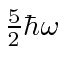01 001(3 perm) 3 310, 02 002(3 perm), 011(3 perm) 6 611, 03 003(3 perm), 210(6 perm), 111 10 1020, 12, 04 004(3), 310(6), 220(3), 211(3) 15 15
The number of states at each energy matches exactly. The parities of the states also match. Remember that the parity is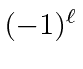for the angular momentum states and that it isfor the Cartesian states. If we were more industrious, we could verify that the wavefunctions in spherical coordinates are just linear combinations of the solutions in Cartesian coordinates.

Jim Branson 2013-04-22